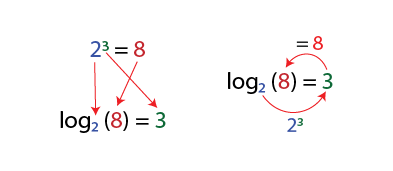#### Cents, powers and logarithms

Cents are based on the mathematical concept of logarithms. Logarithms were introduced by Scottish mathematician John Napier (1550 / 4-1617). The name comes from logos (ratio) and arithmós (number). Logarithms are related to powers.

Two to the third power or $$2^3$$ equals 1 x 2 x 2 x 2:

$$2^3 = 1 * 2 * 2 * 2 = 8$$

Two to the fourth power:

$$2^4 = 1 * 2 * 2 * 2 * 2 = 16$$

A confusing example that shows why we should start with 1:

$$2^0 = 1$$ (1 multiplied by no 2)

After understanding the powers, the following image illustrates its relationship with logarithms:Two, raised to the third power is equal to 8 ( $$2 ^ 3 = 8$$ ). The logarithm indicates the power to which we must raise 2 to obtain 8. The second line reads: base 2 logarithm of 8 = 3. In other words: to what power do we have to raise 2 (base 2) to obtain 8? The answer is 3.

In music, we use base 2 logarithms because octaves use this base naturally. To find out the frequency of a note at an octave distance, we must multiply the frequency of the first note by two. Starting from a C2 with 60 Hz. We obtain the frequency of C3 in this way:

$C3 = 60 * 2 = 120$

To get to C4, we multiply by 2, twice:

$C4 = 60 * 2 * 2 = 240$

Or we can use powers:

$C4 = 60 * 2^2 = 60 * 4 = 240$

The exponent used is related to the number of octaves:

 Note Octaves Power Frequency $C2$ 0 0$2^0 = 1$ 60 Hz. $60 * 2^0 = 60 * 1 = 60$ $C3$ +1 1$2^1 = 2$ 120 Hz.$C3 = C2 * 2^1 = 60 * 2 = 120$ $C4$ +2 2$2^2 = 4$ 240 Hz.$C4 = C2 * 2^2 = 60 * 4 = 240$ $C5$ +3 3$2^3 = 8$ 480 Hz.$C5 = C2 * 2^3 = 60 * 8 = 480$

Let's now use logarithms:

• Let's use the frequencies of C5 (480 Hz.) and C3 (120 Hz.). The notes are at two-octave distance.
• We divide the frequencies and the result is 4:

$$480 / 120 = 4$$

• What is the logarithm base 2 of 4, or what power must we raise 2 to get 4? The answer is 2, just as the number of octaves.

$$log_2(4) = 2$$

• If we multiply C2's frequency $$2^2$$ we get C4's frequency:

$$120 * 2^2 = 480$$

We achieve the same result by subtracting the powers. The power needed to reach C5 from C2 is 3 (see the table above), that of C3 1. If we subtract 3 - 1 we obtain 2. The same result that we obtained with $$log_2 (4) = 2$$.

Unintentionally, we have almost arrived at Ellis's formula to calculate the cents:

we divide the frequencies of the notes and look for the base 2 logarithms of the result.

Only a small detail is missing. We will see that in the next section.

It would help if you went through it many times, with a lot of patience, and you will find your way...

Let's summarize the mathematical concepts learned:

Powers: Logarithms: $$\color{red}2 \color{blue}^3 \color{black}= 1 * 2 * 2 * 2 = \color{green}8$$ Multiply 1 by 2 three times $$log\color{red}_2 \color{black} ( \color{green}8 \color{black} ) = \color{blue}3$$ At what power should we raise 2 to get 8?

If you can go from powers to logarithms and vice versa, you may consider the concept understood!